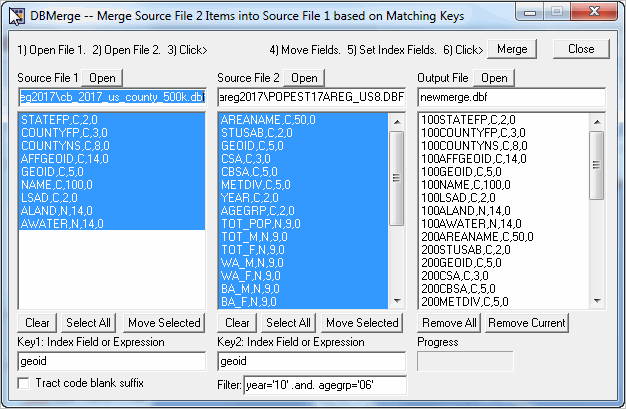﻿ AREG2017

# AREG2017

Mapping Statistical Data -- http://proximityone.com/mapping-statistical-data.htm

AREG2017 -- U.S. by County Population Estimates 2010-2017 by Age-Race/Ethnicity-Gender

See related section http://proximityone.com/areg.htmyear='10' .and. agegrp='06'

C:\msd\areg2017\POPEST17AREG_US8.DBF

c:\areg2017\cb_2017_us_county_500k.dbf

YEAR variable is as follows:

1 = 4/1/2010 Census population

2 = 4/1/2010 population estimates base

3 = 7/1/2010 population estimate

4 = 7/1/2011 population estimate

5 = 7/1/2012 population estimate

6 = 7/1/2013 population estimate

7 = 7/1/2014 population estimate

8 = 7/1/2015 population estimate

9 = 7/1/2016 population estimate

10 = 7/1/2017 population estimate

AGEGRP is as follows:

0 = Total

1 = Age 0 to 4 years

2 = Age 5 to 9 years

3 = Age 10 to 14 years

4 = Age 15 to 19 years

5 = Age 20 to 24 years

6 = Age 25 to 29 years

7 = Age 30 to 34 years

8 = Age 35 to 39 years

9 = Age 40 to 44 years

10 = Age 45 to 49 years

11 = Age 50 to 54 years

12 = Age 55 to 59 years

13 = Age 60 to 64 years

14 = Age 65 to 69 years

15 = Age 70 to 74 years

16 = Age 75 to 79 years

17 = Age 80 to 84 years

18 = Age 85 years or older

ProximityOne -- resources to create and apply insights © ProximityOne